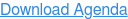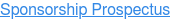# AI & Emerging Tech for Finance

November 2020 | New York Citytrue true true true true true true true true true true true true true true true true true true true true true true true true true true true true true true true true true true true true true true true true true true true true true true true true true true true true true true true true true true true true true true true true true true true true true true true true true true true true true true true true true true true true true true true true true true true true true true true true true true true true true true true true true true true true true true true true true true true true true true true true true true true true